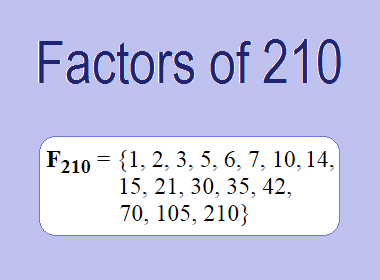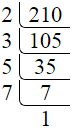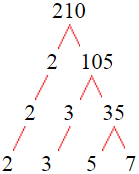# Factors of 210The factors of 210 are 1, 2, 3, 5, 6, 7, 10, 14, 15, 21, 30, 35, 42, 70, 105, and 210 i.e. F210 = {1, 2, 3, 5, 6, 7, 10, 14, 15, 21, 30, 35, 42, 70, 105, 210}. The factors of 210 are all the numbers that can divide 210 without leaving a remainder.

We can check if these numbers are factors of 210 by dividing 210 by each of them. If the result is a whole number, then the number is a factor of 210. Let's do this for each of the numbers listed above:

·        1 is a factor of 210 because 210 divided by 1 is 210.

·        2 is a factor of 210 because 210 divided by 2 is 105.

·        3 is a factor of 210 because 210 divided by 3 is 70.

·        5 is a factor of 210 because 210 divided by 5 is 42.

·        6 is a factor of 210 because 210 divided by 6 is 35.

·        7 is a factor of 210 because 210 divided by 7 is 30.

·        10 is a factor of 210 because 210 divided by 10 is 21.

·        14 is a factor of 210 because 210 divided by 14 is 15.

·        15 is a factor of 210 because 210 divided by 15 is 14.

·        21 is a factor of 210 because 210 divided by 21 is 10.

·        30 is a factor of 210 because 210 divided by 30 is 7.

·        35 is a factor of 210 because 210 divided by 35 is 6.

·        42 is a factor of 210 because 210 divided by 42 is 5.

·        70 is a factor of 210 because 210 divided by 70 is 3.

·        105 is a factor of 210 because 210 divided by 105 is 2.

·        210 is a factor of 210 because 210 divided by 210 is 1.

How to Find Factors of 210?

1 and the number itself are the factors of every number. So, 1 and 210 are two factors of 210. To find the other factors of 210, we can start by dividing 210 by the numbers between 1 and 210. If we divide 210 by 2, we get a remainder of 0. Therefore, 2 is a factor of 210. If we divide 210 by 3, we get a remainder of 0. Therefore, 3 is also a factor of 210.

Next, we can check if 4 is a factor of 210. If we divide 210 by 4, we get a remainder of 2. Therefore, 4 is not a factor of 210. We can continue this process for all the possible factors of 210.

Through this process, we can find that the factors of 210 are 1, 2, 3, 5, 6, 7, 10, 14, 15, 21, 30, 35, 42, 70, 105, and 210. These are the only numbers that can divide 210 without leaving a remainder.

********************

********************

Properties of the Factors of 210

The factors of 210 have some interesting properties. One of the properties is that the sum of the factors of 210 is equal to 576. We can see this by adding all the factors of 210 together:

1 + 2 + 3 + 5 + 6 + 7 + 10 + 14 + 15 + 21 + 30 + 35 + 42 + 70 + 105 + 210 = 576

Another property of the factors of 210 is that the prime factors of 210 are 2, 3, 5, and 7 only.

Applications of the Factors of 210

The factors of 210 have several applications in mathematics. One of the applications is in finding the highest common factor (HCF) of two or more numbers. The HCF is the largest factor that two or more numbers have in common. For example, to find the HCF of 210 and 84, we need to find the factors of both numbers and identify the largest factor they have in common. The factors of 210 are 1, 2, 3, 5, 6, 7, 10, 14, 15, 21, 30, 35, 42, 70, 105, and 210. The factors of 84 are 1, 2, 3, 4, 6, 7, 12, 14, 21, 28, 42, and 84. The largest factor that they have in common is 42. Therefore, the HCF of 210 and 84 is 42.

Another application of the factors of 210 is in prime factorization. Prime factorization is the process of expressing a number as the product of its prime factors. The prime factors of 210 are 2, 3, 5, and 7 since these are the only prime numbers that can divide 210 without leaving a remainder. Therefore, we can express 210 as:

210 = 2 × 3 × 5 × 7

We can do prime factorization by division and factor tree method also. Here is the prime factorization of 210 by division method,210 = 2 × 3 × 5 × 7

Here is the prime factorization of 210 by the factor tree method,210 = 2 × 3 × 5 × 7

Conclusion

The factors of 210 are the numbers that can divide 210 without leaving a remainder. The factors of 210 are 1, 2, 3, 5, 6, 7, 10, 14, 15, 21, 30, 35, 42, 70, 105, and 210. The factors of 210 have some interesting properties, such as having a sum of 576. The factors of 210 have several applications in mathematics, such as finding the highest common factor and prime factorization.